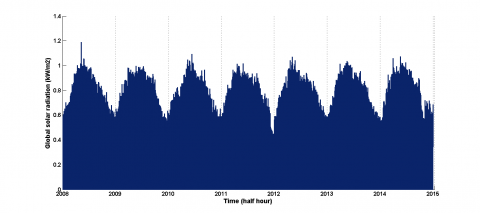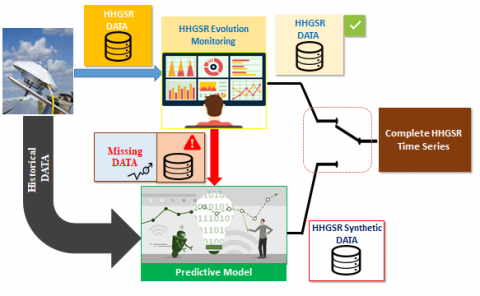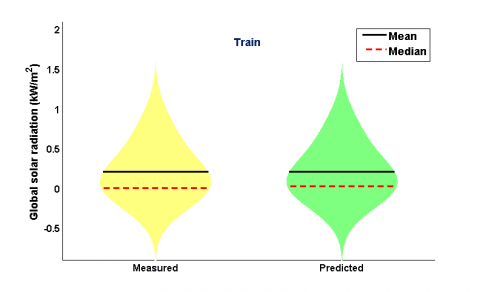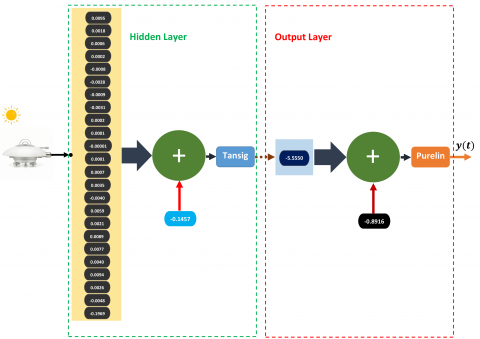# AI Data Driven Approach-Based Endogenous Inputs for Global Solar Radiation Forecasting

AI Data Driven Approach-Based Endogenous Inputs for Global Solar Radiation Forecasting

Mohammed Ali JallalAbdessalam El Yassini Samira Chabaa Abdelouhab Zeroual Saida Ibnyaich

Physics department, Faculty of Sciences Semlalia, Cadi Ayyad University, Marrakesh 40000, Morocco

Industrial Engineering Department, National School of Applied Sciences, Ibn Zohr University, Agadir 80000, Morocco

Corresponding Author Email:
mohammedali.jallal@edu.uca.ac.ma
Page:
27-34
|
DOI:
https://doi.org/10.18280/isi.250104
16 November 2019
|
Accepted:
3 January 2020
|
Published:
29 February 2020
| Citation

OPEN ACCESS

Abstract:

Controlling the random nature of renewable energy sources such as solar radiation at ground, allows electric grid operators to better integrate it. In this paper, an intelligent data-driven model based on artificial neural network with autoregressive input sequence is developed to forecast the global solar radiation (GSR) time series on a half hour resolution in the site of Agdal, Marrakesh, Morocco. The database that is used to create this model was divided into two subsets. The first subset is used for training the proposed model on the data measured during the year 2008 by adopting three efficient optimizers (levenberg-marquardt, resilient backpropagation, and scaled conjugate gradient). The second subset is used for testing the efficiency and the robustness of the developed model to generate accurate predictions during the next six years (from 2009 to 2014). The obtained results demonstrate the accuracy and the stability of the proposed data-driven model to perform prediction in case of GSR measurements intermittence or sensor damage.

Keywords:

artificial neural network, time series, global solar radiation, autoregressive, prediction

1. Introduction

Solar energy is considered as the most effective and economic alternative energy resource. It is considered as one of the most significant clean and renewable energy sources. Designing, controlling, and managing these systems depend highly on the measurements of global solar radiation (GSR) time series. For this raison, many models have appeared to handle this problem, among of them artificial neural networks (ANNs) . ANNs known an explosive growth since the 1980 in several engineering applications such as, meteorological prediction [2-4], image processing , pattern recognition , medical fields , video processing , speech processing , and so on.

To solve the problems related to the scarceness or even the absence of the global solar radiation parameter, several studies have focused on reconstructing the GSR time series using different techniques.

Jallal et al.  proposed a new data-driven model based on the hybridization of artificial neural network with multi-model approach to estimate the hourly global solar radiation time series. Sun et al.  proposed a predictive machine-learning algorithm based on support vector regression that decomposes, clusters, and then forecasts the solar radiation time series. Bakker el al.  adopted different forecasting approaches (parametric regression, quantile regression, quantile regression neural network, random forests, and gradient boosting decision trees) to predict solar radiation measurements. Xue et al.  applied particle swarm optimization and genetic algorithm to regulate the ANN’s parameters to forecast daily diffuse solar radiation data using a set of exogenous inputs.

The majority of the developed GSR predictive models in the literature present some gaps, such as:

1. Most of the GSR time series predictive models are based on different meteorological data (air temperature, precipitation, humidity…) that need more sensors to be employed, which is very expensive;
2. Most of the proposed approaches present a large number of parameters to be optimized and a set of steps to be performed to generate predictions, which increase the training process time of the predictive model;
3. The inputs features selection techniques are needed to determine which exogenous inputs are relevant to generate predictions, which penalizes hardly the training process time.

As a solution for the previous mentioned gaps, the prime objective of this paper is to build an accurate, fast, and robust data-driven-based ANN to predict GSR data in half hour resolution by using only the historical measurements of GSR parameter as exogenous inputs without any need to other meteorological data.

The key elements of innovation of the present paper are the following:

1. An AI data-driven approach with a fewer number of parameters to be optimized is proposed in order to make the training process faster;
2. The accuracy and the stability of the proposed technique are evaluated to forecast the chaotic behavior of the GSR time series;
3. The developed data-driven model presents a high efficiency to infer the relationship between the endogenous inputs and the next GSR value based only on the GSR data recording during the year 2008;
4. The proposed technique shows a high accuracy to forecast the GSR time series during the next six years (from 2009 to 2014);
5. The proposed approach achieved a superior forecasting performance for GSR time series compared to the other predictive techniques.
2. Artificial Neural Network Model

Artificial neural network (ANN), which is analogue the computer systems inspired from studies on the human brain, is considered as a new approach for information processing by learning this information and making it available to use in order to solve efficiently several engineering problems. Such as, classification , control  and so on. However, neural networks are generally used to analyze particular types of problems among them prediction. The precision of the prediction depends on many factors, such as the quantity and the relevance of the input data .

In the literature, neural networks have found great success in modeling and predicting the GSR time series at full time scale (hourly, daily or even monthly) and for several localities in the world [15-17].

2.1 Model of a formal neuron

A formal neuron is a nonlinear function that generate an output signal, which is depend on the setting parameters called synaptic weights and biases. Figure 1 shows a graphic representation of a formal neuron model .

The formal neuron is described in Figure 1.

## 1.pngFigure 1. Formal neuron model

The following pair of equations approximate the neuron’s output:

$u_{i}=\sum_{j=1}^{n} \omega_{i j} x_{j}$  (1)

$h_{i}=\psi\left(u_{i}+b_{i}\right)$  (2)

where,

n is the number of input signals;

$x_{j}$ is the $j t h$ input’s signal;

$\omega_{i j}$ are the synaptic weights;

$\psi$ is the activation function;

$h_{i}$ is the $i t h$ neuron’s output signal;

$b_{i}$ is the neuron’s bias.

2.2 Architecture of the artificial neural network

The most common neural network model is the multilayer neural network. This type of neural network is known as a supervised learning algorithm that involves a desired output to train their parameters. The notation $I-H 1-H 2-O$ refers to a multilayer neural network with two hidden layers. The first layer have I  neurons is called the input layer, the middle layers are the hidden layers with $H 1, H 2$ neurons, where their outputs are calculated by Eq. (3) and Eq. (4), respectively. The last is the output layer equipped with O neurons, which is computed by Eq. (5) . The Figure 2 depicts a neural network without a feedback reaction with two hidden layers.

## 2.pngFigure 2. Architectural diagram of a multilayer neural network with two hidden layers

$h_{1, j}=\psi\left(\sum_{i=1}^{I} \omega_{i j} x_{i}+b_{1, j}\right)$  (3)

$h_{2, m}=\psi\left(\sum_{j=1}^{H 1} \omega_{j m} h_{1, j}+b_{2, m}\right)$  (4)

$y_{k}=\varphi\left(\sum_{m=1}^{H 2} \omega_{m k} h_{2, m}+b_{3, k}\right)$  (5)

where,

$\psi$ is the hidden layers activation function;

$\varphi$  is the output layer activation function;

$\omega_{i j}$  are the weights between the input and the first hidden layer;

$\omega_{j m}$  are the weights between the first and the second hidden layer;

$\omega_{m k}$  are the weights between the second hidden layer and the output layer;

$b_{1, j}$  are the biases of the first hidden layer;

$b_{2, m}$  are the biases of the second hidden layer;

$b_{3, k}$  are the biases of the output layer;

$x_{i}$  are the inputs of the ANN model;

$h_{1, j}$  are the outputs of the first hidden layer;

$h_{2, m}$  are the outputs of the second hidden layer;

$y_{k}$  are the outputs of the ANN model.

After defining the ANN architecture, an efficient optimizer needs to be applied in order to adjust the ANN parameters (wiegths and biases). In the present study, three efficient backpropagation (BP) optimizers (levenberg-marquardt, resilient backpropagation, and scaled conjugate gradient), were adopted to train the ANN parameters . The BP optimizers are based on computing the gradient of the ANN output error $e$ over the network’s weights and biases.

The prime objective of the ANN training process is to minimize the error function $e$ expressed as follows:

$e=\sum_{k=1}^{K} \sum_{i=1}^{N}\left(y_{k, i}-\hat{y}_{k, i}\right)^{2}$  (6)

where,

K is the number of outputs;

N is the length of output vectors;

$y_{k, i}$ is the $i t h$ pattern of the $k t h$ desired output;

$\widehat{y}_{k, i}$ is the $k t h$ pattern of the $k t h$ ANN’s predicted output.

Then, the gradient values are applied for tuning the ANN’s parameters using the following updating formula :

$w_{i j}(I t+1)=\mathbf{w}_{i j}(\mathrm{It})+\mu \Delta w_{i j}(I t)$  (7)

with

$\Delta w_{i j}(I t)=\frac{\partial e(I t)}{\partial w_{i j}(I t)}$  (8)

where,

$I t$ is the current iteration;

$\mu$ is the learning rate of the ANN model.

3. Database Description and Visualization

The database used in the present study is constructed from the GSR measurements at the weather station, Agdal, Marrakesh, Morocco. These measurements were recorded each half hour from sun set to sun rise during 7 years, from 2008 to 2014, with (t = 1, ..., 122736).

In Figure 3, the GSR parameter evolution during seven years was depicted.

## 3.pngFigure 3. Half hour evolution of the GSR time series at Agdal, Marrakesh, Morocco during the period 2008 to 2014

4. Results and Discussion

4.1 Development of the GSR predictive model

In this study, an intelligent data-driven model is proposed to forecast the next value of GSR parameter using 24 endogenous inputs of the same parameter that is recorded in the past 24 half hour. The developed model is applied to monitor the GSR time series and generate an accurate synthetic data of this parameter when missing data are detected.

The description of the proposed approach to forecast the GSR data is defined in Figure 4.

The first option to create the proposed model is related to the distribution of the database. The first subset of GSR data measured during the year 2008 are used for training the network’s parameters and the second subset is used for testing the efficiency and the robustness of the developed model to generate accurate predictions during the next six years (from 2009 to 2014).

To design the proposed data-driven-based ANN model a number of steps need to be followed (Figure 5).

To demonstrate the efficiency and the robustness of the developed model, the number of hidden layers, number of neurons in the hidden layer, activation functions, and training algorithms need to be determined. The optimal ANN structure is the one that minimize the mean squared error (MSE) defined by the following equation :

$M S E=\frac{1}{N} \sum_{i, j=1}^{N}\left(Y_{j}-Y_{i}\right)^{2}$  (9)

where,

$Y_{j}$ is the ANN model output;

$Y_{i}$ is the target (the desired output);

N is the number of samples.

## 4.pngFigure 4. Architecture of the proposed approach

## 5.pngFigure 5. Basic flowchart for artificial neural network design

4.2 Number of hidden layers

Before inspecting the effect of the hidden layer’s number on the prediction precision, the LM optimizer and the tangent activation function were adopted by the initial ANN model.

In Table 1, the effect of increasing the hidden layer number was investigated. The obtained values of the MSE demonstrate that the best choice is to use a single hidden layer, where the increase of hidden layers’ number increases the computing burden without any gain in performance.

Table 1. Performance of the predictive model in terms of the MSE indicator during the training and the testing phases based on the number of hidden layers

 Number of hidden layers Train MSE Test MSE 1 2 3 2.27 10-3 2.32 10-3 2.42 10-3 2.35 10-3 2.44 10-3 2.57 10-3

In the following sub-section, the developed ANN model uses a single hidden layer as an optimal choice, and then the effect of the activation functions is examined.

4.3 Selection of the best activation function

To find out the corresponding activation function for the hidden layer’s neurons, the ANN structure was evaluated by different activation functions.

The determination of an appropriate ANN activation function play a key role to improve the prediction accuracy.

Several activation functions were adopted by the ANN neurons , among them are:

• Linear activation function (purelin),
• Log-sigmoid activation function (logsig),
• Hyperbolic or bipolar tangent activation function (tansig),

The results presented in Table 2 demonstrate the performance of the hyperbolic tangent activation function (Tansig) to perform accurate GSR predictions. Then, this activation function was adopted to approach the inputs’ non-linearity.

For the output layer a linear activation function was adopted in order to overcome the divergence of the developed model to predict the target (GSR).

In the subsequent sub-section, the number of neurons in the single hidden layer’s ANN is studied.

Table 2. Performance of the hidden layer’s activation function in terms of the MSE indicator during the training and the testing phases

 Hidden layer activation function Train MSE Test MSE Logsig Tansig Radbas 2.31 10-3 2.27 10-3 2.28 10-3 2.39 10-3 2.35 10-3 2.38 10-3

4.4 Number of neurons in the hidden layer

To define the best number of neurons in the hidden layer there is no deterministic approach that can determine efficiently this number. For this reason, the number of neurons in the hidden layer was increased from the value 1 and the MSE values that are correspond to each hidden layer’s neurons are recorded during the training and the testing phases. The best neuron number is the one that minimize the MSE indicator.

From the results depicted in Figure 6, the best hidden neurons number n that minimize the MSE indicator and the computing time during the training and the testing phases is $n=1$.

## 6.pngFigure 6. Training and testing MSE values versus the number of hidden neurons

In the next sub-section, the performance of three BP algorithms was evaluated in order to adjust efficiently the developed model’s parameters.

4.5 Selection of the training algorithm

There are various backpropagation algorithms, in this study, three training algorithms are used, which present a good performance in different field of research, such as scaled conjugate gradient (SCG), levenberg-marquardt (LM) and resilient backpropagation (RP) .

The graphs below shows the evolution of the training MSE values versus the number of iterations for the three optimizers.

From the attained values of MSE during the training phase that are depicted in Figure 7, the LM algorithm is the most performant one to train the synaptic weights and biases of the developed ANN’s structure with the best convergence time. Then, this algorithm is applied to train the final ANN’s configuration.

## 7.pngFigure 7. Training and testing MSE evolution versus the number of iterations

4.6 Performance synthesis of the developed model

To present the performance results of the developed data-driven model, the scattering diagram accompanied with the correlation coefficient (R) is applied in order to determine the relationship between the measured and the predicted GSR time series during the training and the testing phase.

The scattering diagrams results that are presented in Figure 8 demonstrate the accuracy and the stability of the proposed approach to forecast efficiently the GSR time series during the training and the testing phases.

Based on the correlation coefficient (R) values given by Figure 8, the developed data driven model achieved a precision of 99.12% to map the relationship between the endogenous inputs and the next GSR values in the training process based on the measurements of the GSR time series recorded in the year 2008. Besides, a prediction accuracy of 99.13% is achieved in the testing phase to forecast the GSR data during the next six years (from 2009 to 2014).

Figure 9 compares Violin plots of the measured versus the predicted time series during the training and the testing phases.

From Figure 9, we notice a good agreement between the measured data and the developed model in the training phase.

Based on the attained results of these graphs (Figure 9), the proposed data-driven model forecast the target accurately, where the predicted time series has approximately the same mean, median, and shape compared to the measured GSR time series.

## 8a.png(a)

## 8b.png(b)

Figure 8. Scattering diagrams during the training (a) and the testing (b) phases

## 9a.png(a)

## 9b.png(b)

Figure 9. Violin plots during the training (a) and the testing (b) phases

In Table 3, the performance of the proposed AI data driven approach was compared with previous related works in terms of the correlation coefficient (R). It can be observed from the presented results in Table 3 that the proposed approach achieved a superior forecasting performance for GSR time series compared to the other predictive techniques.

Overall results demonstrate the high performance of the proposed model to be implemented in Agdal weather station of the city of Marrakesh, Morocco, to generate accurate GSR predictions in case of intermittence of their measurements or sensor damage.

Figure 10 gives the identified structure of the proposed predictive model based on the LM optimizer.

## 10.pngFigure 10. Identified structure of the proposed data-driven model

Table 3. Validation of the proposed model against well-established predictive models on solar radiation forecasting

 References Study location Applied techniques R (%)  (2018)  (2018)    (2018)    (2018)    (2019)  (2019)  (2020)  (2020)  (2020) Present study (2020) Toledo, Spain Adrar, Algeria   Tamanrasset, Algeria   Ghardaia, Algeria   Adana, Turkey Antakya, Turkey İstanbul, Turkey Tekirdağ, Turkey Muğla, Turkey Marrakesh, Morocco SVR-PLS Empirical model Empirical model Empirical model DENFIS DENFIS PVGIS HELIOSAT HELIOSAT Proposed approach 93.3 96.7   92.9   82.5   94.2 97.9 97.1 97.2 97.6 99.13
5. Conclusion

In this study, an intelligent data-driven model is proposed to forecast the next value of GSR parameter using 24 endogenous inputs of the same parameter that is recorded in the past 24 half hour. The developed model demonstrate a high accuracy and stability to monitor the GSR time series in the half hour resolution and proves its efficiency to generate an accurate synthetic data of this parameter when missing data are detected or in the case of sensor damage without any need to other meteorological data.

The proposed AI data-driven approach achieved a high accuracy to approach the chaotic behavior of the GSR time series with a few numbers of parameter to be optimized. This feature makes the training process faster and the model more stable to perform predictions.

Future researches will focus on two aspects: (1) the prediction generated by the developed model will be used for monitoring the energy production of a solar energy system; (2) the integration of some new metaheuristic algorithms with the present model will be studied.

Acknowledgment

The meteorological database used for this study was funded by the joint international laboratory “Remote Sensing of Water Resources in Semi-Arid Mediterranean Areas.”

References

 Jallal, M.A., Chabaa, S., Zeroual, A. (2020). A new Artificial Multi-Neural Approach to estimate the hourly global solar radiation in a semi-arid climate site. Theoretical and Applied Climatology, 139: 1261-76. https://doi.org/10.1007/s00704-019-03033-1

 Jallal, M.A., Chabaa, S., El Yassini, A., Zeroual, A., Ibnyaich, S. (2019). Air temperature forecasting using artificial neural networks with delayed exogenous input, 2019 Int. Conf. Wirel. Technol. Embed. Intell. Syst. (WITS), Fez, Morocco, pp. 1-6. https://doi.org/10.1109/WITS.2019.8723699

 Do Nascimento Camelo, H., Sérgio Lucio, P., Verçosa Leal Junior, J., Von Glehn dos Santos, D., Cesar Marques de Carvalho, P. (2018). Innovative hybrid modeling of wind speed prediction involving time-series models and artificial neural networks. Atmosphere, 9(2): 77. https://doi.org/10.3390/atmos9020077

 Ahmad, A., Anderson, T.N., Lie, T.T. (2015). Hourly global solar irradiation forecasting for New Zealand. Solar Energy, 122: 1398-1408. https://doi.org/10.1016/j.solener.2015.10.055

 Cheng, P.M., Tejura, T.K., Tran, K.N., Whang, G. (2017). Detection of high-grade small bowel obstruction on conventional radiography with convolutional neural networks. Abdominal Radiology, 43: 1120-1127. https://doi.org/10.1007/s00261-017-1294-1

 Schmidhuber, J. (2015). Deep Learning in neural networks: An overview. Neural Networks, 61: 85-117. https://doi.org/10.1016/j.neunet.2014.09.003

 Da Silva, G.L.F., Valente, T.L.A., Silva, A.C., De Paiva, A.C., Gattass, M. (2018). Convolutional neural network-based PSO for lung nodule false positive reduction on CT images. Computer Methods and Programs in Biomedicine, 162: 109-118. https://doi.org/10.1016/j.cmpb.2018.05.006

 Karpathy, A., Toderici, G., Shetty, S., Leung, T., Sukthankar, R., Li, F.F. (2014). Large-scale video classification with convolutional neural networks. 2014 IEEE Conference on Computer Vision and Pattern Recognition, Columbus, OH, USA, pp. 1725-1732. https://doi.org/10.1109/CVPR.2014.223

 Goldberg, Y. (2015). A primer on neural network models for natural language processing. Journal of ArtificialIntelligence Research, 57. https://doi.org/10.1613/jair.4992

 Sun, S., Wang, S., Zhang, G., Zheng, J. (2018). A decomposition-clustering-ensemble learning approach for solar radiation forecasting. Solar Energy, 163: 189-199. https://doi.org/10.1016/j.solener.2018.02.006

 Bakker, K., Whan, K., Knap, W., Schmeits, M. (2019). Comparison of statistical post-processing methods for probabilistic NWP forecasts of solar radiation. Solar Energy, 191: 138-150. https://doi.org/10.1016/j.solener.2019.08.044

 Xue, X. (2017). Prediction of daily diffuse solar radiation using artificial neural networks. International Journal of Hydrogen Energy, 42(47): 28214-28221. https://doi.org/10.1016/j.ijhydene.2017.09.150

 Kocadagli, O., Langari, R. (2017). Classification of EEG signals for epileptic seizures using hybrid artificial neural networks based wavelet transforms and fuzzy relations. Expert Systems with Applications, 88: 419-434. https://doi.org/10.1016/j.eswa.2017.07.020

 Khajeh Talkhoncheh, M., Shahrokhi, M., Askari, M.R. (2017). Observer-Based adaptive neural network controller for uncertain nonlinear systems with unknown control directions subject to input time delay and saturation. Information Sciences, 418-419: 717-737. https://doi.org/10.1016/j.ins.2017.08.024

 Lopes, F.M., Silva, H.G., Salgado, R., Cavaco, A., Canhoto, P., Collares-Pereira, M. (2018). Short-term forecasts of GHI and DNI for solar energy systems operation: assessment of the ECMWF integrated forecasting system in southern Portugal. Solar Energy, 170: 14-30. https://doi.org/10.1016/j.solener.2018.05.039

 Ghimire, S., Deo, R.C., Downs, N.J., Raj, N. (2019). Global solar radiation prediction by ANN integrated with European Centre for medium range weather forecast fields in solar rich cities of Queensland Journal of Cleaner Production, 216: 288-310. https://doi.org/10.1016/j.jclepro.2019.01.158

 Mubiru, J. (2008). Predicting total solar irradiation values using artificial neural networks. Renewable Energy, 33(10): 2329-2332. https://doi.org/10.1016/j.renene.2008.01.009

 Jallal, M.A., Chabaa, S., Zeroual, A. (2020). A novel deep neural network based on randomly occurring distributed delayed PSO algorithm for monitoring the energy produced by four dual-axis solar trackers. Renewable Energy, 149: 1182-1196. https://doi.org/10.1016/j.renene.2019.10.117

 Al-Shamisi, M.H., Assi, A.H., Hejase, H.A.N. (2013). Artificial neural networks for predicting global solar radiation in al ain city – UAE. International Journal of Green Energy, 10: 443-456. https://doi.org/10.1080/15435075.2011.641187

 García-Hinde, O., Terrén-Serrano, G., Hombrados-Herrera, M., Gómez-Verdejo, V., Jiménez-Fernández, S., Casanova-Mateo, C., Sanz-Justo, J., Martínez-Ramón, M., Salcedo-Sanz, S. (2018). Evaluation of dimensionality reduction methods applied to numerical weather models for solar radiation forecasting. Engineering Applications of Artificial Intelligence, 69: 157–167. https://doi.org/10.1016/j.engappai.2017.12.003

 Bailek, N., Bouchouicha, K., Al-mostafa, Z., El-shimy, M. (2018). A new empirical model for forecasting the diffuse solar radiation over Sahara in the Algerian Big South. Renewable Energy, 117: 530-537. https://doi.org/10.1016/j.renene.2017.10.081

 Kisi, O., Heddam, S., Mundher, Z. (2019). The implementation of univariable scheme-based air temperature for solar radiation prediction: New development of dynamic evolving neural-fuzzy inference system model. Applied Energy, 241: 184-195. https://doi.org/10.1016/j.apenergy.2019.03.089

 Ener Rusen, S., Konuralp, A. (2020). Quality control of diffuse solar radiation component with satellite-based estimation methods. Renewable Energy, 145: 1772-1779. https://doi.org/10.1016/j.renene.2019.07.085